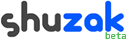TeamworkSign in

 Owner: Joe Members: 19

` `
Surface Fitting Algorithm - 21 June, 2007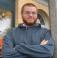Joe says Hello everyone, I have to fit a surface to data. I'm thinking that a polynomial in x and y with cross terms would suffice (i.e. nothing like exponentials, logs). Would least squares be the easiest way to implement this? I've already pretty much finished an algorithm that does this, but it seems to take forever. This is most likely due to the fact that the input file is over 4 million lines long. Total Topic Karma: 0 - More by this Author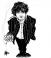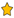+0 Karma
Now I am no graduate student. Hell I am just starting college this fall. But that DOES seem like it would be a BIT long.
- Author's History - 22 June, 2007+0 Karma
The input file is long or the code would be?

The input file is that long because I'm fitting a surface to a 2048x2048 CCD plate. So thats 2048*2048 pixels and each pixel has its own value. It adds up.

If you meant the code would be long, it isn't really. The algorithm I made already generates a matrix in one loop. Then takes two more loops to get it in row reduced echelon form.
- Author's History - 22 June, 2007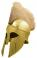+0 Karma
Depends alot on the Algorithm you wrote.
Calculate the time complexity of your algorithm and try to find a way to help your computer, like reducing number of variables and so on. Does your input file really needs to be that long?
- Author's History - 27 June, 2007+0 Karma
I think that my algorithm is pretty streamlined as far as I can tell. And yes my input file has to be that long. There is no getting around that. These are 2048x2048 images and each pixel has a value.

I did, however, find the use of the -O2 (that's an "oh") with gcc. When doing a fourth order fit it cut the time from five minutes to under two!
- Author's History - 27 June, 2007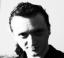+0 Karma
I would appreciate if you post some line of pseudo-code or else. I'm working on a thesis on Algorithms so I find problems like that fascinating :-)
- Author's History - 27 June, 2007+0 Karma
I can give you the whole thing if you want. I apologize for lack of comments.

[code=default]
```
#include <stdio.h>
#include <math.h>
double tothepower(double base, double exp);
int main(int argc, char *argv[])
{
double val, exp;
int i, j, k, kk,flag,midflag,ii,fit;
char line;
FILE *in, *out;
fit=4;
double xtx[(fit+1)*(fit+1)][(fit+1)*(fit+1)+1];
in=fopen(argv,"r");
k=0;
\\ Set everything to zero.
for(i=0;i<fit*fit;i++)
{
for(j=0;j<fit*fit+1;j++)
{
xtx[i][j]=0;
}
}
xtx[i][j]=0;
while (fgets(line,50,in) != NULL)
{
val=1;
sscanf(line,"%lf %lf %lf",&val,&val,&val);
\\ Initialize the array.
for (i=0;i<fit*fit;i++)
{
for(j=0;j<fit*fit;j++)
{
exp=(i-i%fit)/fit;
exp=i%fit;
exp=(j-j%fit)/fit;
exp=j%fit;
if (exp+exp<fit)
{
if(exp+exp<fit)
{
xtx[i][j]=xtx[i][j]+tothepower(val,exp)*tothepower(val,exp)*tothepower(val,exp)*tothepower(val,exp);
}
}
}
}
for  (i=0;i<fit*fit;i++)
{
exp=(i-i%fit)/fit;
exp=i%fit;
if(exp+exp<fit)
{
xtx[i][fit*fit]=xtx[i][fit*fit]+tothepower(val,exp)*tothepower(val,exp)*val;
}
}
}
\\ Make matrix upper triangular
for(ii=0;ii<fit*fit;ii++)
{
if (xtx[ii][ii]!=0)
{
for(j=fit*fit;j>=ii;j--)
{
xtx[ii][j]=xtx[ii][j]/xtx[ii][ii];
for(i=ii+1;i<fit*fit;i++)
{
xtx[i][j]=xtx[i][j]-xtx[i][ii]*xtx[ii][j];
}
}
}
}
\\ Make matrix identity
for(ii=fit*fit-2;ii>=0;ii--)
{
for(i=ii;i>=0;i--)
{
for(j=fit*fit;j>=ii+1;j--)
{
xtx[i][j]=xtx[i][j]-xtx[ii+1][j]*xtx[i][ii+1];
}
}
}
out=fopen(argv,"w");
\\ Output best fit parameters.
for(i=0;i<fit*fit;i++)
{
fprintf(out,"%25.23e\n",xtx[i][fit*fit]);
}
}
double tothepower(double base, double exp)
{
int carpow=1;
double carry=1;
while (carpow<=exp)
{
carry=carry*base;
carpow++;
}
return carry;
}```
[/code]
- Author's History - 27 June, 2007+0 Karma
This code looks so similar to Matlab code!
In what language is it C++ ?
- Author's History - 30 July, 2007
Comment:

 Name: